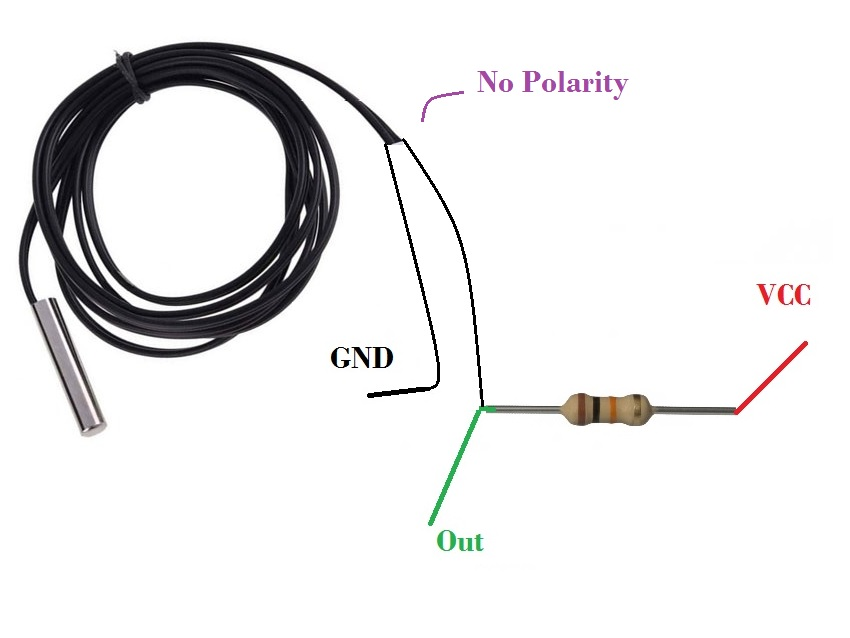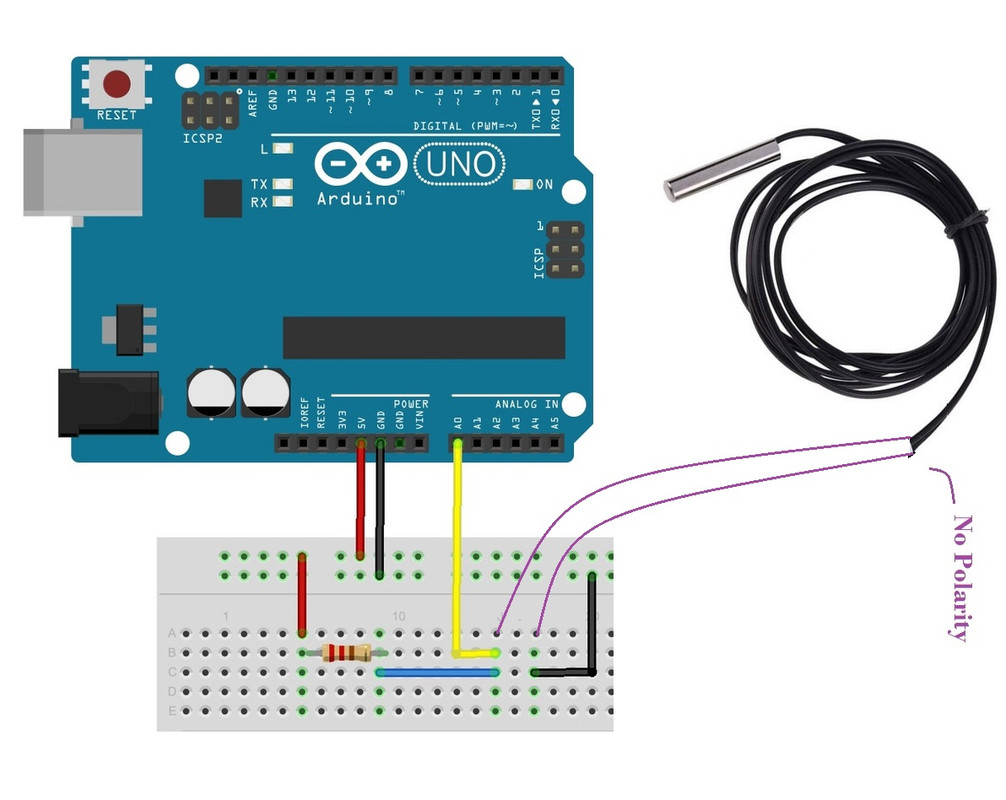1

### Description

The NTC 10K Waterproof Probe Analog Temperature Sensor is a sensor Based on the thermistor's resistance with a 0.5m cable, the cable comes with a 2-pin connector you can always cut the connecter and work with the ordinary cable, it can calculate the ambient temperature it has an operating Voltage of 5V and the temperature measurement range is from -20 to 105 ° C with measurement Accuracy of ±0.5°C. compatible with well-liked electronic hardware platforms like the Arduino and ESP32 and some thermostat solutions.

Package Includes:

• 1x NTC Temperature Analog Sensor NTC 10K Waterproof Probe

Features:

• Longevity of service, high reliability
• Compatible with some thermostat solutions
• 0.5m cable, the cable comes with a 2-pin connector you can always cut the connecter and work with the ordinary cable
• Small size, powerful, strong capability of surge current protection
• Instant response to surge current
• Wide operation range
• Big material constant (B value), low remain resistance.

Description:

The NTC 10K Waterproof Probe Analog Temperature Sensor is a sensor Based on the thermistor's resistance with a 0.5m cable, the cable comes with a 2-pin connector you can always cut the connecter and work with the ordinary cable, it can calculate the ambient temperature it has an operating Voltage of 5V and the temperature measurement range is from -20 to 105 ° C with measurement Accuracy of ±0.5°C. compatible with well-liked electronic hardware platforms like the Arduino and ESP32. Thermistors are straightforward and reasonably priced. The use of thermistors would be best in remote weather stations, home automation systems, and equipment control and protection circuits. The code is relatively straightforward because they are analog sensors as opposed to digital temperature sensors, which call for complex libraries and a lot of code.

Principle of Work:

The resistance of thermometers, which are variable resistors, varies with temperature. They are categorized based on how they react to temperature changes in terms of resistance. Resistance decreases as temperature rises in thermistors with a negative temperature coefficient (NTC). The most popular thermistors are NTC ones. NTC thermistors are created by heating and compressing a semiconducting material (like metal oxide or ceramic) to create a temperature-sensitive conducting material. Charge carriers in the conducting material enable current to pass through it. The semiconducting material releases more charge carriers when the temperature is elevated. Electrons are the charge carriers in ferric oxide-based NTC thermistors. Electron holes serve as the charge carriers in nickel oxide NTC thermistors.

Types of Thermistor

Depending on its resistance variation with respect to the surrounding temperature, there are two types of thermistors. They are explained in detail below:-

1. PTC – Positive Temperature Coefficient.

Its resistance is directly proportional to the temperature i.e., its resistance decreases with a decrease in temperature and vice-versa.

2. NTC - Negative Temperature Coefficient.

Its resistance is indirectly proportional to the temperature i.e., its resistance decreases with an increase in temperature and vice-versa.

We are using an NTC thermistor in our application. 103 is indicating the resistance of the thermistor at normal temperature means 10k Ohm.

To measure the resistance of the NTC Thermistor, first, we will measure the voltage from the voltage divider which is the output. And the voltage divider equation is as follows.
Vout = Vin*[R2/(R1+R2)]
Since we know Vin, R1, and Vout we can calculate the value of the NTC thermistor R2 with the following equation

R2=(Vout*R1) / (Vin-Vout)

Pinout of the Board:

This cable can be plugged directly into some thermostat solutions or you can cut the connecter and use it as a normal NTC sensor.

You can connect the NTC Cable directly to your circuit you need to use the voltage divider method so you will need a 10k resistor and the pinout will going to be like the next pic:NTC 10K Waterproof Probe Description
Resistor side VCC
Middle Out
the other side of the NTC Cable GND

Applications:

1. Used in household air conditioners, car air conditioners, refrigerators, freezers, water heaters, water dispensers, heaters, dishwashers, Temperature measurement and control in cabinets, washing machines, dryers, medium and low temperature drying ovens, constant temperature ovens, and other occasions.

Circuit:

Middle to pin A0 on Arduino

the other side of the NTC to GND on Arduino

Resistor side to 5V on ArduinoLibrary:

you don't need any library to work with this sensor.

Code:

This code is going to print the Temperature Sensor on the Serial Monitor.

```#define ntc_pin A0 // Pin,to which the voltage divider is connected
#define vd_power_pin 2 // 5V for the voltage divider
#define nominal_resistance 10000 //Nominal resistance at 25⁰C
#define nominal_temeprature 25 // temperature for nominal resistance (almost always 25⁰ C)
#define samplingrate 5 // Number of samples
#define beta 3950 // The beta coefficient or the B value of the thermistor (usually 3000-4000) check the datasheet for the accurate value.
#define Rref 10000 //Value of resistor used for the voltage divider
int samples = 0; //array to store the samples
void setup(void) {
pinMode(vd_power_pin, OUTPUT);
Serial.begin(9600); //initialize serial communication at a baud rate of 9600
}
void loop(void) {
uint8_t i;
float average;
samples = 0;
// take voltage readings from the voltage divider
digitalWrite(vd_power_pin, HIGH);
for (i = 0; i < samplingrate; i++) {
delay(10);
}
digitalWrite(vd_power_pin, LOW);
average = 0;
average = samples / samplingrate;
Serial.println("\n \n");
Serial.println(average);
// Calculate NTC resistance
average = 1023 / average - 1;
average = Rref / average;
Serial.print("Thermistor resistance ");
Serial.println(average);
float temperature;
temperature = average / nominal_resistance; // (R/Ro)
temperature = log(temperature); // ln(R/Ro)
temperature /= beta; // 1/B * ln(R/Ro)
temperature += 1.0 / (nominal_temeprature + 273.15); // + (1/To)
temperature = 1.0 / temperature; // Invert
temperature -= 273.15; // convert absolute temp to C
Serial.print("Temperature ");
Serial.print(temperature);
Serial.println(" *C");
delay(2000);
}
```

Technical Details:

• Line length: 0.5M
• Probe size: 5x25mm
• Output: 2 lines
• Type: NTC 10k±1%3950
• Measuring range: -20 to 105 ° C
• B constant: 3380 k - / + 1%
• Typical constant dissipation 5 MW / °C
• Insulated probe: > 100 MOhm
• Maintain pressure: 9.8N (1kgF) for 1 minute without deformation
• Peak hold voltage-time: 2 seconds, AC1800V 1mA 2 seconds

Resources:

Datasheet

Tutorial

Comparisons:
thermometers are very cheap, but imprecise, and their resistance changes with temperature in a non-linear manner, determining the temperature requires a little "work" on your software's part. Although more expensive, active devices like the LM35 are more accurate and provide a linear voltage-vs-temperature characteristic, making it simpler to determine the temperature. The LM35 is calibrated. The thermistor has to be calibrated against some known-good temperature standards to provide a temperature measurement in a range from -20 to 105 ° C. The LM35 device does not require any external calibration or trimming to provide typical accuracies of ±¼°C at room temperature and ±¾ °Cover a full −55°C to 150°C temperature range.